# 了解赫夫曼树一文就够了

## 一. 定义

1. 路径：从树中一个结点到另一个结点之间的分支构成两个结点之间
的路径。

2. 路径长度：路径的上分支的数目。就是从一个结点到另一个结点有多少个路径

3. 结点的路径长度：从到该结点的路径长度。

4. 树的路径长度：从树根到每一个结点的路径长度之和。

5. 结点的权：在一些应用中，赋予树中结点的一个有某种意义实数

6. 结点的带权路径长度：从根结点到各个叶结点的路径长度与相应结点权值的乘积。

7. 树的带权路径长度：所有叶结点的带权路径长度之和，记作：
W P L = ∑ k = 1 n W k l k （ 其 中 ， n ： 叶 结 点 数 目 ； W k ： 叶 结 点 k 的 权 值 ； l k 根 到 k 之 间 的 路 径 长 度 ） {WPL= \sum_{k=1}^n W_kl_k} （其中，n：叶结点数目；W_k：叶结点 k的权值；l_k根到k之间的路径长度）

8. 最优二叉树/ 赫 夫曼树： 假设有n个权值(w1,w2…wn)，试
构造一棵有n个叶子结点的二叉树，每个叶子结点带权为wi ，
则其中带权路径长度WPL最小的二叉树称作最优二叉树或赫
夫曼树。

## 二. 赫夫曼算法

#### 1.基本思想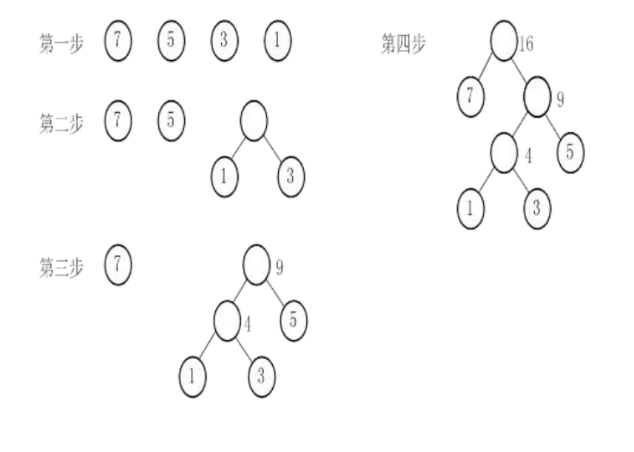#### 2.算法的实现

（1）赫夫曼树的存储结构

​ 权值 双亲 左孩子 右孩子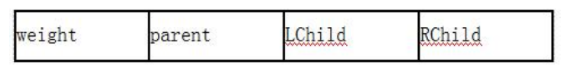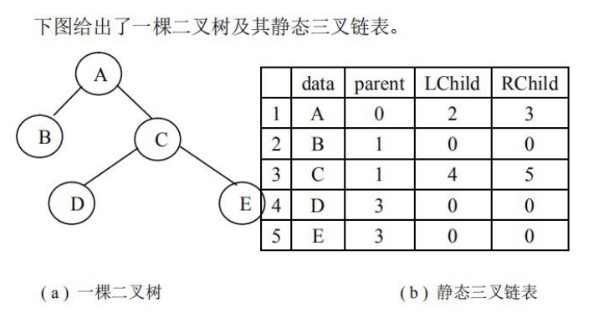（2）赫夫曼树的类型定义

#define N 20 /* 叶子结点的个数。*/
#define M 2*N-1 /* 所有结点的个数。*/
typedef struct
{
int weight ; /* 结点的权值*/
int parent ; /* 双亲的下标*/
int LChild ; /* 左孩子结点的下标*/
int RChild ; /* 右孩子结点的下标*/
} HTNode, HuffmanTree[M+1];
/* HuffmanTree 是一个结构数组类型，0 号单元不用。 */
void CrtHuffmanTree(HuffmanTree ht, int w[ ], int n)
{    /*构造哈夫曼树 ht[M+1]， w[ ]存放 n 个权值。*/
/* 1 ~ n 号单元存放叶子结点，初始化*/
for(i=1;i<=n;i++) ht[i] ={ w[i],0,0,0};
m=2*n-1;
for(i=n+1;i<=m;i++) ht[i] ={0,0,0,0};
/* n+1 ~ m 号单元存放非叶结点，初始化 */
——————————初始化完毕！对应算法步骤 1，下面左边的表——————
for(i=n+1; i<=m; i++) /*创建非叶结点，建哈夫曼树*/
{
select(ht, i-1, s1, s2);
/* 在 ht ~ ht[i-1] 的范围内选择两
个 parent 为 0 且 weight 最小的结点，其序号分别赋值给 s1、s2 返回 */
ht [i].weight= ht [s1].weight+ ht [s2].weight;
ht [s1].parent=i; ht [s2].parent=i;
ht [i].LChild=s1; ht [i].RChild=s2;
} /*哈夫曼树建立完毕*/
}


（3）代码事列：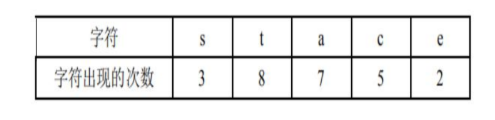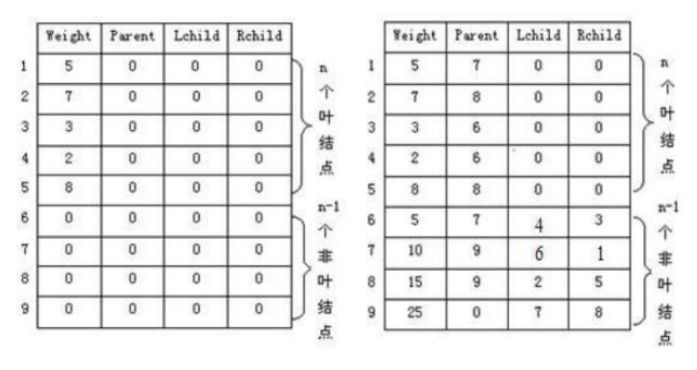## 三.赫夫曼编码

#### 1.编码和解码（考例题）

（1）数据压缩过程称为编码。即将文件中的每个字符均转

（2）数据解压过程称为解码。即将二进制位串转换为对应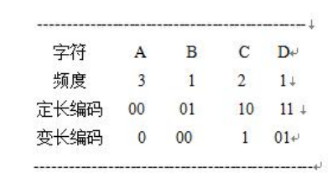，例如传送过去的字符串中前4个字符的子串’0000’就可有多种译法，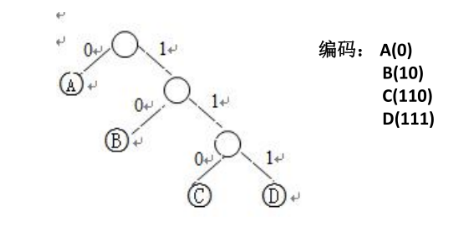#### 2.编码实现

//一一一一赫夫曼树和赫夫曼编码的存储表示----------
typedef struct{
unsigned int weight;
unsigned int parent, lchild, rchild;
}EINode, * HuffmanTree: //动态分配数组存储赫夫曼树
typedef char * *HuffmanCode; //动态分配数组存储赫夫曼编码表
void HaffmanCoding(HuffmanTree &HT, HuffmanCode &HC，int *w，int n) {
//  w存放n个字符的权值(均>0)，构造赫夫曼树HT,并求出n个字符的赫夫曼编码HC
/---构造赫夫曼树HT---
if (n<= 1) return;//如果只有一个结点，就没必要构造了
m = 2 * n - 1; //结点总数
HT = (HuffmanTree)malloc((m+ 1) * sizeof(HTNode)); //0号单元未用
for (p=HT, i=1; i<=n; ++i, ++p, ++w) *p = {*w,0, 0,0 );
for(; i≤=m; ++i，++p) *p ={0,0,0.0};
for (i=n+ 1; i<=m; ++i) { //建赫夫曼树
//在HT[1..i-1]选择parent 为0且weight 最小的两个结点,其序号分别为s1s2.
Select(HT, i- 1, sl, s2);
HT[sl]. parent = i; HT[s2]. parent = i;
HT[i]. Ichild = s1; HT[i].rchild = s2;
HT[i].weight = HT[s1].weight + HT[s2].weight;
}
/---从叶子到根逆向求每个字符的赫夫曼编码---
HC= (HuffmanCode)malloc ((n+1)*sizeof(char *)); //分配n个字符编码的头指针向量
cd = (char * )malloc(n* sizeof(char)); //分配求编码的工作空间
cd[n-1] = "\0"; //编码结束符。
for(i=1; i<=n; ++i) { //逐个字符求赫夫曼编码
start = n-1; //编码结束符位置
for (c=i, f= HT[i]. parent; f!=0; :c= f, f=HT[f].parent) //从叶子到根逆向求编码
if (HT[f].lchild==c) cd[--start] = "0”;
else cd[--start] ="1";
HC[i] = (char *)malloc((n-start)*sizeof(char)); // 为第i个字符编码分配空间
strcpy(HC[i], &cd[start]); //从cd复制编码(串)到HC
}
free(ed); //释放工作空间
}//HuffanCoding


## 四.边学边练

（1）分析这三棵树路径长度和带权路径长度那个最小有什么特点。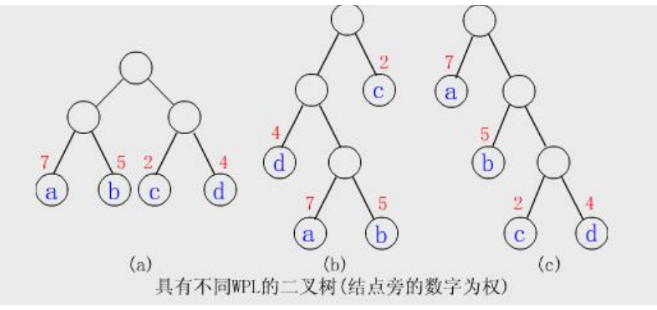(a)：WPL＝72+52+22+42=36 树的路径长度：10

(b)：WPL= 73+53+21+42=46 树的路径长度：12

（2） 给定8个权值：5，29，7，8，14，23，3，11，构造赫夫曼树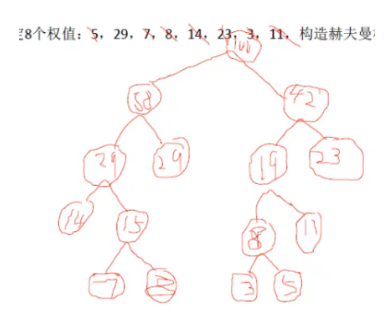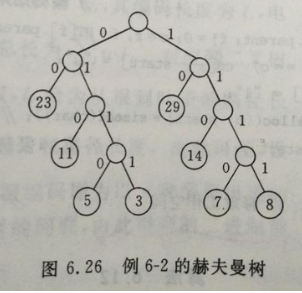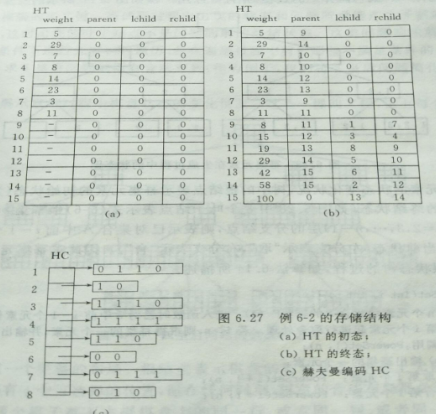12-17
06-0512-10
08-17598
07-03205
04-201810
11-09760
05-096806
11-0564
01-1882
07-08833
08-24564
04-26770
06-093079
11-152147